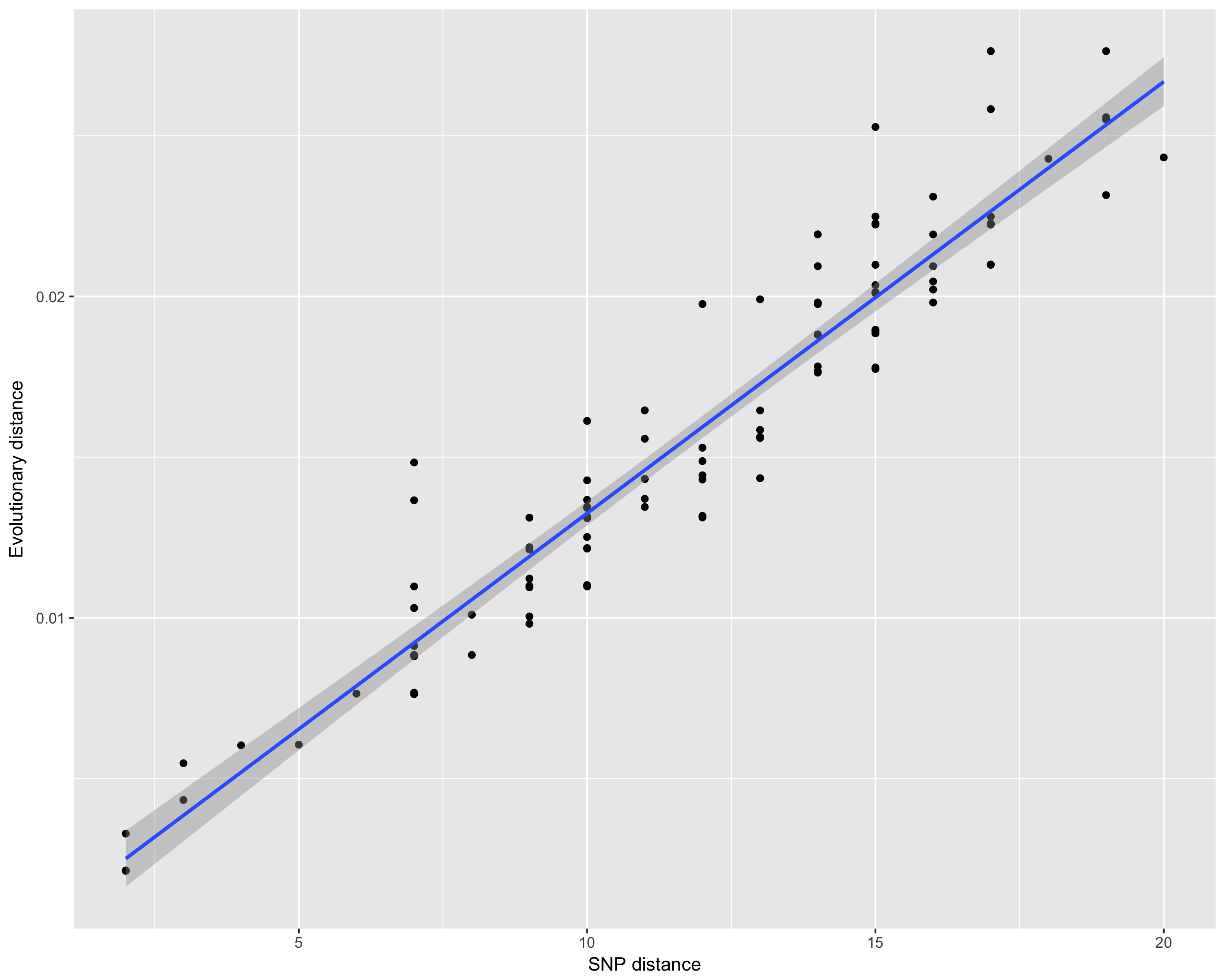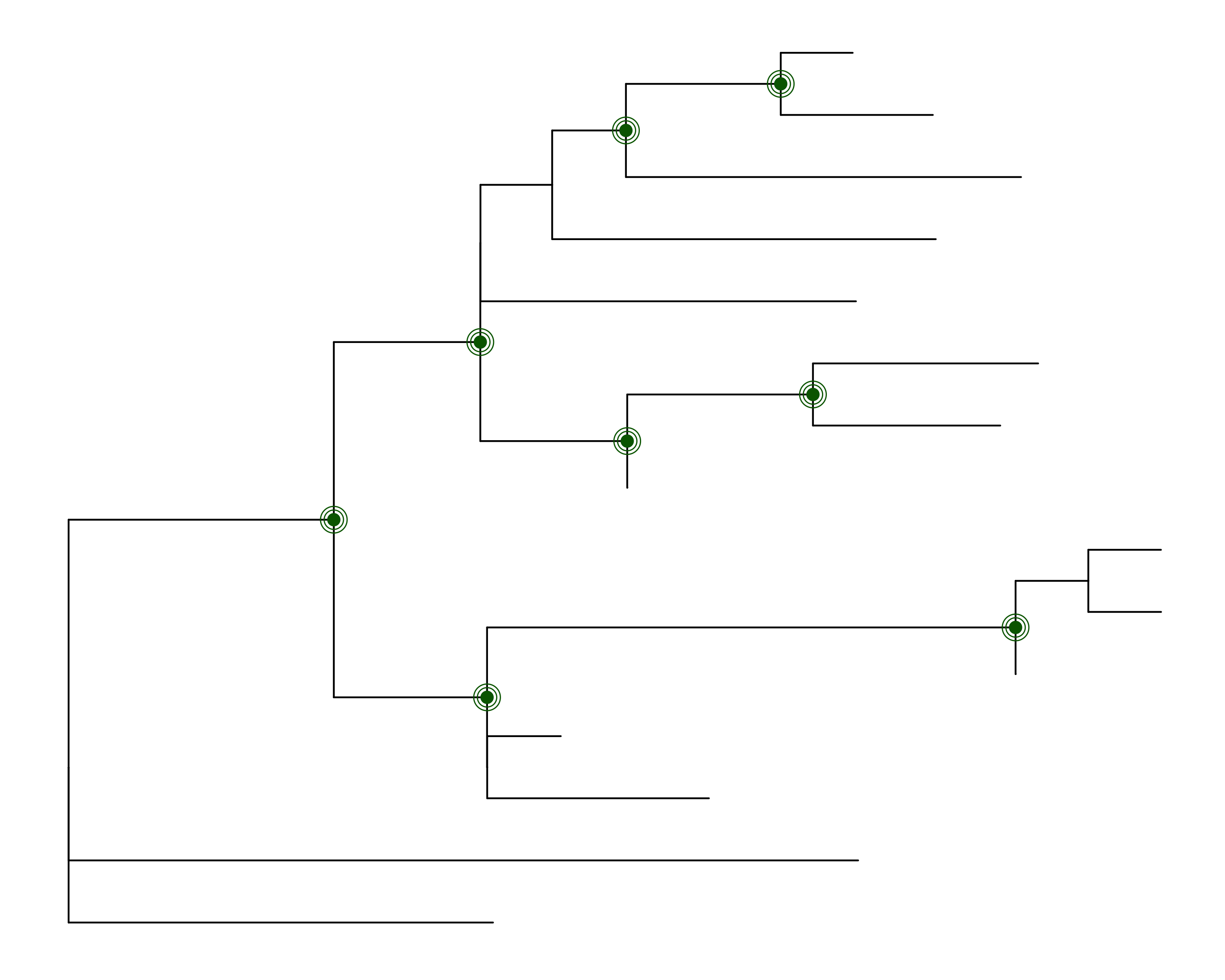# `harrietr`: An `R` package for various phylogenetic and evolutionary biology data manipulations

## Why `harrietr`:

Harriet is believed to be Charles Darwin’s pet giant turtle. It is thought that Harriet spent the latter part of her life at in Brisbane, Australia. Thus, Harriet satisfies the three criteria to name this package: (1) it is somehow evolutionarily related; (2) it has an Australian connection; and (3) it avoids the prefix `phylo` used by many `R` evolutionarily- relavant packages. The appended `r` just helps differentiate to make it easier to search in Google, and aludes to the fact that it is related to the programming language `R`.

## How to get it

### From CRAN:

1. Add `Bioconductor` to your list of default repositories:

`setRepositories(ind = 1:2)`

2. Install `harrietr`:

`install.packages("harrietr", dependencies = TRUE)`

### Latest and gratest version from GitHub:

You must use `devtools`:

1. If you don’t have `devtools` installed:

`install.packages('devtools')`

2. Add `Bioconductor` to your list of default repositories:

`setRepositories(ind = 1:2)`

3. Install `harrietr`:

`devtools::install_github("andersgs/harrietr")`

## How to use it

Three functions are provided at this time:

`dist_long` — This function takes as input an alignment in `DNA.bin` format calculates all the pairwise distances, and returns all the unique pairwise distances as a `data.frame` in a long format. For example, in the case of three samples:

id1 id2 distance
sample1 sample2 dist_12
sample1 sample3 dist_13
sample2 sample3 dist_23

You can give `dist_long` a tree (object of class `phylo`), and it will add a fourth column with the pairwise distance obtained from the tree:

id1 id2 distance evol_dist
sample1 sample2 dist_12 evol_dist_12
sample1 sample3 dist_13 evol_dist_13
sample2 sample3 dist_23 evol_dist_23

`melt_dist` — This function is used by `dist_long`, but it takes as input a distance matrix. This might be useful if you alredy have a distance matrix that is imported into `R`.

`get_node_support` — This function is written to work with trees generated by IQTREE. In particular, if the tree was generated when calculating node support by both ultrafast bootstrap and SH approximate likelihood ratio test, IQTREE writes the support as the node label in the Newick file in the following format: `"SH-aLRT/uBS"`. In other words, it is a string with two values separated by a `slash`. The first value is the `SH-aLRT` support (as a percentage) and the second value is the ultrafast bootstrap support (also as a percentage).

The output is a `data.frame` with each row representing an internal node, with information that can be used to plot support information layers on a tree.

## Some use cases

### Comparing distances

Assume you have a tree, and you want to understand what is the relationship between the branch lengths and the number of SNPs. The function `dist_long` can help you get there:

``````library(harrietr)
library(ggplot2)
data("woodmouse")
data("woodmouse_iqtree")
dist_df <- dist_long(aln = woodmouse, order = woodmouse_iqtree\$tip.label, dist = "N", tree = woodmouse_iqtree)
ggplot(dist_df, aes(x = dist, y =  evol_dist)) +
geom_point() + stat_smooth(method = 'lm') +
ylab("Evolutionary distance") +
xlab("SNP distance")``````

This will produce the following image:### Indicating nodes that have support on a tree

Assume you have generated your ML tree with IQTREE, and wish to plot it in `R`, and indicate which nodes have 50% or more support values for both metrics (note: the value of 50% is likely too low, these values are chosen only for illustration purposes). The function `get_node_support` can help you get there:

``````library(ggtree)
library(dplyr)
library(harrietr)
data("woodmouse_iqtree")
p1 <- ggtree(woodmouse_iqtree)
node_support <- get_node_support(woodmouse_iqtree)
p1 +
geom_point(data = node_support %>% dplyr::filter(`SH-aLRT` >= 50 & uBS >= 50), aes(x = x, y = y), colour = 'darkgreen', size = 3) +
geom_point(data = node_support %>% dplyr::filter(`SH-aLRT` >= 50 & uBS >= 50), aes(x = x, y = y), colour = 'darkgreen', size = 5, pch = 21) +
geom_point(data = node_support %>% dplyr::filter(`SH-aLRT` >= 50 & uBS >= 50), aes(x = x, y = y), colour = 'darkgreen', size = 7, pch = 21)``````

This will produce the following image:### Getting group level statistics

Assume you have classified your samples into different groups (say A, B, and C). These could be anything (e.g., MLST, sample source, host, etc.), and you want summary information among and between the groups (e.g., IQR, min/max dist). You can use `dist_long` and `add_metadata` to generate the `data.frame` you need:

``````library(ggplot2)
library(dplyr)
library(harrietr)
data("woodmouse")
data("woodmouse_iqtree")
data("woodmouse_meta")
dist_df <- dist_long(aln = woodmouse, order = woodmouse_iqtree\$tip.label, dist = "N", tree = woodmouse_iqtree)
dist_df <- add_metadata(dist_df, woodmouse_meta, isolate = 'SAMPLE_ID', group = 'CLUSTER', remove_ind = TRUE)
dist_df %>%
dplyr::group_by(CLUSTER) %>%
dplyr::summarise(q50 = median(dist),
q25 = quantile(dist, prob = c(0.25)),
q75 = quantile(dist, prob = c(0.75)),
min_dist = min(dist),
max_dist = max(dist)) %>%
ggplot( aes( x = CLUSTER, y = q50)) +
geom_errorbar( aes(ymin = q25, ymax = q75),width = 0.25 ) +
geom_point(size = 3, colour = 'darkred') +
geom_point( aes( y = min_dist), colour = 'darkgreen', size = 3) +
geom_point( aes( y = max_dist), colour = 'darkgreen', size = 3) +
ylab("Pairwise SNP difference") +
xlab("Groups")``````

This will produce the following image: